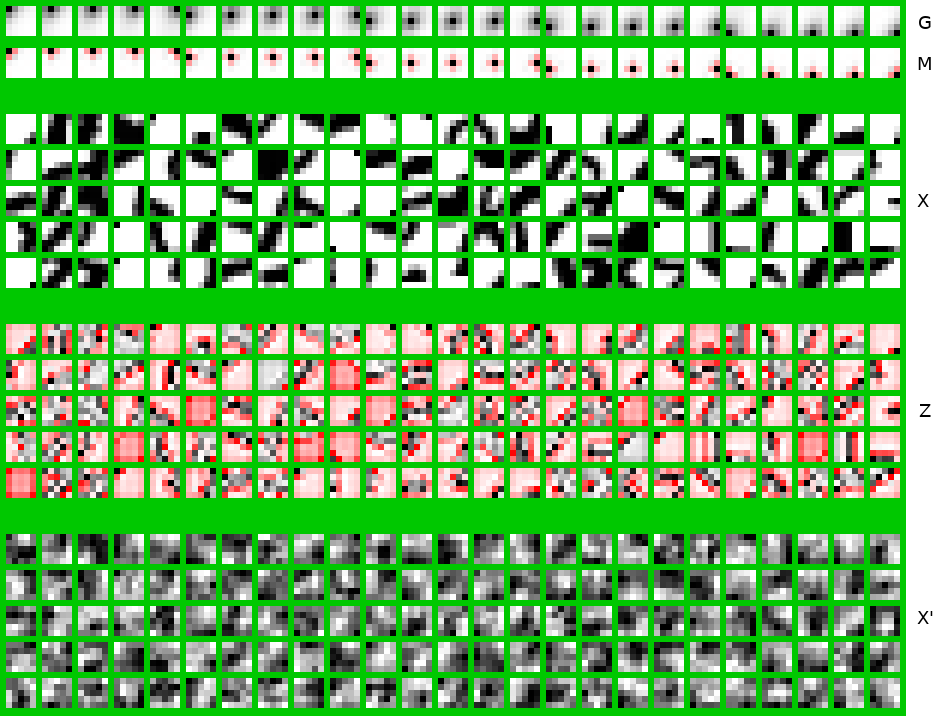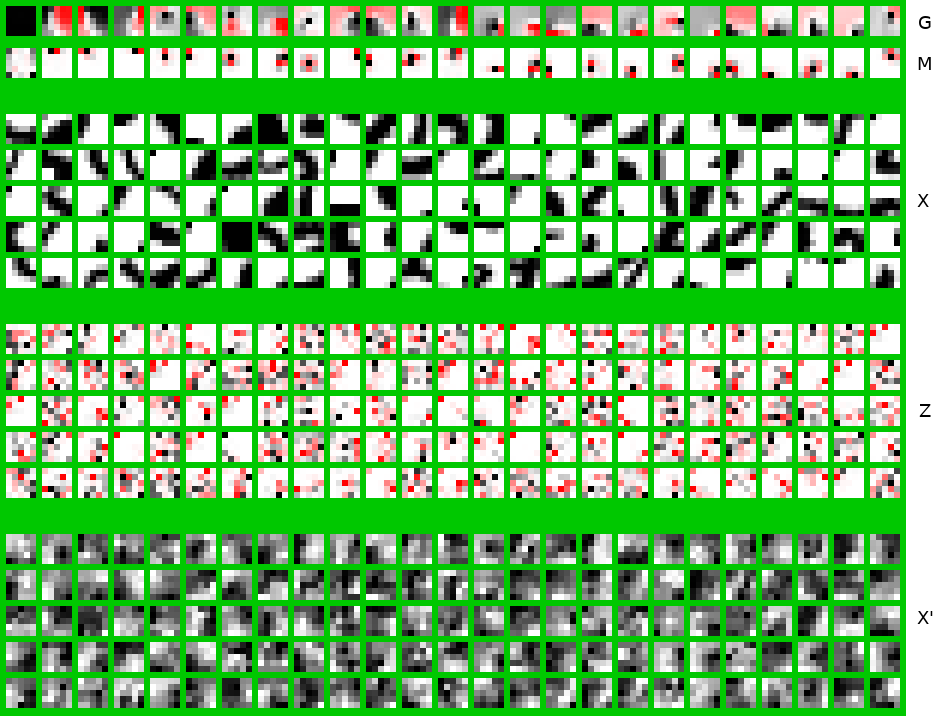[<< | Prev | Index | Next | >>]

## Saturday, September 01, 2018

#### Simple PDM Test on Mnist Patches

[Research log]

Coded up a quick test training 5x5 patches of Mnist* using equations 8 and 11 of my Aug 14 post. I initialized them to identity (M=I, Z=X) both to encourage some order in the final result and because I was curious to see how it would diverge from a perfect orthogonal encoding.

Here is using a normal prior (equation 8):Here is using a Laplace prior (equation 11):White is zero. Black is positive, red is negative.

M is the encoding (perception) matrix. G is the synthesis matrix, aka the inverse of M. X is an example batch of input patches from mnist. Z is the corresponding encodings after multiplying by M. X' are randomly generated patches drawn from the corresponding prior (normal or Laplace) and passed through G.

None of this is novel, really, since this simple linear case has been studied ad nauseum decades ago. Nonetheless, interesting to verify the Laplace prior leads to somewhat sparser Z, oriented features, and perhaps marginally more structured X's?

Most interesting to me is the illustration of G against M. Those two rows are simply matrix inverses of each other (each patch, if you unrolled it, is a single row or column of the corresponding matrix). Note how in the normal case, the generative patches are essentially Gaussian blotches (presumably equaling the co-variance matrix of the inputs), whereas their inverse, the perception filters, are center-surround wavelets (with multiple ripples).

The Laplace case is even more interesting, in that the generative patches, G, are quite large, yet the perception patches, M, remain relatively compact. In particular one wouldn't easily guess G by looking at M (even though G is exactly the matrix inverse of M). If nothing else this serves as a warning about interpreting the "meaning" of a node or feature (or neuron) based on what it responds to: Here G is more indicative of the meaning (it is what a feature paints onto the canvas), whereas M is what it responds to (and typically what we see in empirical experiments). M in some sense is (merely) the hallmark of G.

[<< | Prev | Index | Next | >>]

Simon Funk / simonfunk@gmail.com